# Samacheer Kalvi 12th Chemistry Solution Chapter 6 Solid State

Tamilnadu State Board Solutions for Class 12th Chemistry Solutions Chapter 6 Solid State Questions and Answers will help you to improve the complete subject knowledge. Every student must look at the single concept included in Samacheer Kalvi 12th Chemistry Solutions Chapter 6 Solid State Book Solutions Answers Guide Solutions PDF. All the concepts are explained clearly with examples and pictures.

Students can easily avoid the struggle to get the best book for Chemistry Solutions Chapter 6 Solid State Book Solutions Answers Guide learning. You have to go through Chapterwise Samacheer Kalvi Class 12th Textbook Solutions for Chemistry Solutions Chapter 6 Solid State Book Solutions for better practice.

## Tamilnadu Samacheer Kalvi 12th Chemistry Solutions Chapter 6 Solid State

Students who wish to have the strong basics of Chemistry Solutions Chapter 6 Solid State can use Tamilnadu State Board Class 12th Chemistry Solutions Chapter 6 Solid State Questions and Answers Guide pdf. Enhance your knowledge by referring to the Samacheer Kalvi Solutions pdf. We provided a free pdf of Tamilnadu State Board Class 12th Chemistry Solutions Chapter 6 Solid State Book Solutions Answers Guide material and textbook for students. Check it out now and start preparing for the exam immediately. Score maximum marks in the exam by referring to Samacheer Kalvi Class 12th Chemistry Solutions Chapter 6 Solid State Book Solutions Answers Guide Solutions Pdf.

### Samacheer Kalvi 12th Chemistry Solid State Text Book Evalution

12th Chemistry Chapter 6 Book Back Answers Question 1.
Graphite and diamond are ………..
(a) Covalent and molecular crystals
(b) ionic and covalent
(c) both covalent crystals
(d) both molecular crystals
(c) both covalent crystals

12th Chemistry Unit 6 Book Back Answers Question 2.
An ionic compound Ax By crystallizes in fee type crystal structure with B ions at the centre of each face and A ion occupying centre of the cube, the correct formula of A B is ………..
(a) AB
(b) AB3
(c) A3B
(d) A8B6
(b) AB3
Hint: Number of A ions = $$\left( \frac { { N }_{ c } }{ 8 } \right)$$ = $$\left( \frac { 8 }{ 8 } \right)$$ = 1
Number of B ions = $$\left( \frac { { N }_{ f } }{ 2 } \right)$$ = $$\left( \frac { 6 }{ 2 } \right)$$ = 3
Simplest formula AB3

12th Chemistry Solid State Guide Pdf Question 3.
The rano of close packed atoms to tetrahedral hole in cubic packing is ………..
(a) 1:1
(b) 1:2
(c) 2:1
(d) 1:4
(b) 1:2
Hint: If number of close packed atoms =N; then, The number of Tetrahedral holes formed = 2N, Number of Octahedral holes formed = N. Therefore N : 2N = 1 : 2

12th Chemistry Solid State Book Back Answers Question 4.
Solid CO2 is an example of ………..
(a) Covalent solid
(b) metallic solid
(c) molecular solid
(d) ionic solid
(c) molecular solid
Hint: Lattice points are occupied by CO2 molecules

Samacheer Kalvi Guru 12th Chemistry Question 5.
Assertion: monoclinic sulphur is an example of monoclinic crystal system.
Reason: for a monoclinic system, a $$\neq$$ b $$\neq$$ c and α = γ = 90° , β $$\neq$$ 90°.
(a) Both assertion and reason are true and reason is the correct explanation of assertion.
(b) Both assertion and reason are true but reason is not the correct explanation of assertion.
(c) Assertion is true but reason is false.
(d) Both assertion and reason are false.
(a) Both assertion and reason are true and reason is the correct explanation of assertion

Samacheer Kalvi 12th Chemistry Guide Question 6.
In calcium fluoride, having the flurite structure the coordination number of Ca2+ ion and F Ion are ………..
(a) 4 and 2
(b) 6 and 6
(c) 8 and 4
(d) 4 and 8
(c) 8 and 4
Hint: CaF2 has cubical close packed arrangement Ca2+ ions are in face centered cubic arrangement, each Ca2+ ions is surrounded by 8 F ions and each F ion is surrounded by 4 Ca2+ ions.Therefore coordination number of Ca2+ is 8 and of F is 4 pattern is (NA is the Avogadro number)

12th Chemistry Lesson 6 Book Back Answers Question 7.
The number of unit cells in 8gm of an element X (atomic mass 40) which crystallizes in bcc pattern is (NA is the Avogadro number)
(a) 6.023 x 1023
(b) 6.023 x 1022
(c) 60.23 x 1023
(d) $$\left( \frac { 6.023\times { 10 }^{ 23 } }{ 8\times 40 } \right)$$
(b) 6.023 x 1022
Hint: In bcc unit cell, 2 atoms = 1 unit cell
Number of atoms in 8g of element is, Number of moles = $$\left( \frac { 8g }{ 40g,{ mol }^{ -1 } } \right)$$ = 0.2 mol
1 mole contains 6.023 x 1023 atoms 0.2 mole contains 0.2 x 6.023 x 1023 atoms
$$(\frac{1unit cell}{2 atoms})$$ x 0.2 x 6.023 x 1023 = 6.023 x 1022 unit cells

Solid State Questions And Answers Pdf Question 8.
The number of carbon atoms per unit cell of diamond is ………..
(a) 8
(b) 6
(c) 1
(d) 4
(a) 8
Hint: In diamond carbon forming fee. Carbon occupies comers and face centres and also occupying half of the tetrahedral voids.
$$\left( \frac { { N }_{ c } }{ 8 } \right)$$ + $$\left( \frac { { N }_{ f } }{ 2 } \right)$$ + 4C atoms in Td voids
$$\left( \frac { 8 }{ 8 } \right)$$ + $$\left( \frac { 6 }{ 2 } \right)$$ + 4 = 8.

Samacheer Kalvi 12th Chemistry Solutions Question 9.
In a solid atom M occupies ccp lattice and $$\left( \frac { 1 }{ 3 } \right)$$ of tetrahedral voids are occupied by atom N. Find the formula of solid formed by M and N.
(a) MN
(b) M3N
(C) MN3
(d) M3N2
(d) M3N2
Hint: If the total number of M atoms is n, then the number of tetrahedral voids = 2 n. Given that $$\left( \frac { 1 }{ 3 } \right)$$rd of tetrahedral voids are occupied i.e., $$\left( \frac { 1 }{ 3 } \right)$$ x 2 n are occupied by N atoms.
∴ M : N ⇒ n : $$\left( \frac { 2 }{ 3 } \right)$$
1 : $$\left( \frac { 1 }{ 3 } \right)$$ 3 : 2 ⇒ M3N2

Solid State Chemistry Class 12 Notes Pdf Question 10.
The Composition of a sample of wurizite is Fe0.93 O1.00 what % of Iron present in the form of Fe3+
(a) 16.05%
(b) 15.05%
(c) 18.05%
(d) 17.05%
(b) 15.05%
Hint: Let the number of Fe2+ ions in the crystal be x. The number of Fe3+ ions in the crystal be y. Total number of Fe2+ and Fe3+ ions is x + y. Given that x + y = 0.93. The total charge= 0 x (2+) + (0.93 – x) (+ 3) – 2 = 0 ⇒ 2x + 2.97 – 3x – 2 = 0 x 0.79 Percentage of Fe3+ = $$\left( \frac { (0.93-0.79) }{ 0.93 } \right)$$100 = 15.05%

Solid State Questions And Answers Question 11.
The ionic radii of A+ and B are 0.98 x 10-10 m and 1.81 x 10-10 m , the coordination number of each ion in AB is ………..
(a) 8
(b) 2
(c) 6
(d) 4
(c) 6
Hint: $$\frac { { r }_{ { c }^{ 1 } } }{ { r }_{ { A }^{ – } } }$$ = $$\left( \frac { 0.98\times { 10 }^{ -10 } }{ 1.81\times { 10 }^{ -10 } } \right)$$ = 0.54. It is in the range of 0.414, 0.732,hence the coordination number of each ion is 6.

Solid State Chemistry Class 12 State Board Question 12.
CsCl has bcc arrangement, its unit cell edge length is 400pm, its inter atomic distance is ………..
(a) 400pm
(b) 800pm
(c) $$g\sqrt { 3 }$$ x 100pm
(d) $$\left( \frac { \sqrt { 3 } }{ 2 } \right)$$ x 400 pm
(d) $$\left( \frac { \sqrt { 3 } }{ 2 } \right)$$ x 400 pm
Hint:
$$g\sqrt { 3 }$$a = rCs+ + 2rCs + rCs+
$$\left( \frac { \sqrt { 3 } }{ 2 } \right)$$a = (rCs+ + rCs)
$$\left( \frac { \sqrt { 3 } }{ 2 } \right)$$400 = inter ionic distance

12th Chemistry Solutions Samacheer Kalvi Question 13.
A solid compound XY has NaCl structure, if the radius of the cation is 100pm , the radius of the anion will be ………..
(a) $$\left( \frac { 100 }{ 0.414 } \right)$$
(b) $$\left( \frac { 0.732 }{ 100 } \right)$$
(c) 100 x 0.414
(d) $$\left( \frac { 0.414 }{ 100 } \right)$$
(a) $$\left( \frac { 100 }{ 0.414 } \right)$$
Hint: For an fcc structure

11th Chemistry Evaluate Yourself Answers Samacheer Question 14.
The vacant space in bcc lattice unit cell is ………..
(a) 48%
(b) 23%
(c) 32%
(d) 26%
(c) 32%
Hint: Packing efficiency = 68%. Therefore empty space percentage = (100-68) = 32%

Samacheer Kalvi 12th Chemistry Book Solutions Question 15.
The radius of an atom is 300pm, if it crystallizes in a face centered cubic lattice, the length of the edge of the unit cell is ………..
(a) 488.5pm
(b) 848.5pm
(c) 884.5pm
(d) 484.5pm
(b) 848.5pm
Hint: Let edge length = a $$g\sqrt { 2 }$$a = 4r
a = $$\left( \frac { 4\times 300 }{ \sqrt { 2 } } \right)$$
a = 600 x 1.414
a = 848.4 pm

Samacheer Kalvi 12 Chemistry Solutions Question 16.
The fraction of total volume occupied by the atoms in a simple cubic is ………..
(a) $$\left( \frac { \pi }{ 4\sqrt { 2 } } \right)$$
(b) $$\left( \frac { \pi }{ 6 } \right)$$
(c) $$\left( \frac { \pi }{ 4 } \right)$$
(d) $$\left( \frac { \pi }{ 3\sqrt { 2 } } \right)$$
(b) $$\left( \frac { \pi }{ 6 } \right)$$
Hint: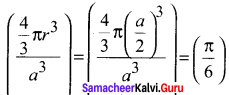Class 12 Samacheer Chemistry Solutions Question 17.
The yellow colour in NaCl crystal is due to ………..
(a) excitation of electrons in F centers
(b) reflection of light from Cl ion on the surface
(c) refraction of light from Na+ ion
(d) all of the above
(a) excitation of electrons in F centers

Samacheer Kalvi 12th Chemistry Guide Pdf Question 18.
If ’a’ stands for the edge length of the cubic system; sc ,bcc, and fcc. Then the ratio of radii of spheres in these systems wilL be respectively.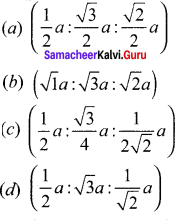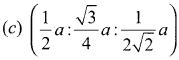Hing: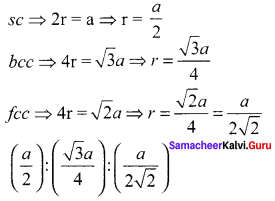12th Samacheer Kalvi Chemistry Guide Question 19.
If a is the length of the side of the cube, the distance between the body centered atom and one comer atom in the cube will be ………..
(a) $$\left( \frac { 2 }{ \sqrt { 3 } } \right) a$$
(b) $$\left( \frac { 4 }{ \sqrt { 3 } } \right) a$$
(c) $$\left( \frac { \sqrt { 3 } }{ 4 } \right) a$$
(d) $$\left( \frac { \sqrt { 3 } }{ 2 } \right) a$$
(d) $$\left( \frac { \sqrt { 3 } }{ 2 } \right) a$$
Hint: If a is the length of the side, then the length of the leading diagonal passing through the body centered atom is $$g\sqrt { 3 }$$a. Required distance = $$\left( \frac { \sqrt { 3 } }{ 2 } \right) a$$

Question 20.
Potassium has a bcc structure with nearest neighbor distance 4.52 A. its atomic weight is 39. Its density will be ………..
(a) 915 kg m-3
(b) 2142 kg m-3
(c) 452 kg m-3
(d) 390 kg m-3
(a) 915 kg m-3
Hint:
(ρ) = $$\frac { nM }{ { a }^{ 3 }{ N }_{ A } }$$For bcc n = 2 M 39. Nearest distance 2r = 4.52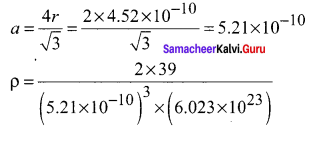ρ = 915 kg m-3 NA

Question 21.
Schottky defect in a crystal is observed when ………..
(a) unequal number of anions and anions are missing from the lattice
(b) equal number of anions and anions are missing from the lattice
(e) an ion leaves its normal site and occupies an interstitial site
(d) no ion is missing from its lattice.
(b) equal number of anions and anions are missing from the lattice

Question 22.
The cation leaves its normal position in the crystal and moves to some interstitial position, the defect in the crystal is known as ………..
(a) Schottky defect
(b) F center
(c) Frenkel defect
(d) non-stoichiometric defect
(c) Frenkel defect

Question 23.
Assertion – due to Frenkel defect, density of the crystalline solid decreases.
Reason – in Frenkel defect cation and anion leaves the crystal.
(a) Both assertion and reason are true and reason is the correct explanation of assertion.
(b) Both assertion and reason are true but reason is not the correct explanation of assertion.
(c) Assertion is true but reason is false.
(d) Both assertion and reason are false
(d) Both assertion and reason are false

Question 24.
The crystal with a metal deficiency defect is ………..
(a) NaCI
(b) FeO
(c) ZnO
(a) KCI
(b) FeO

Question 25.
A two dimensional solid pattern formed by two different atoms X and Y is shown below. The black and white squares represent atoms X and Y respectively. The simplest formula for the compound based on the unit cell from the pattern is ………..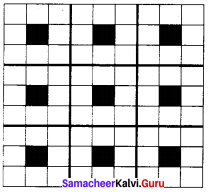(a) XY8
(b) X4Y9
(c) XY2
(d) XY4
(a) XY8

Question 1.
Define unit cell.
A basic repeating structural unit of a crystalline solid is called a unit ccli.

Question 2.
Cive any three characteristics of ionic crystals.

1. Ionic solids have high melting points.
2. These solids do not conduct electricity, because the ions are fixed in their lattice positions.
3. They do conduct electricity in molten state (or) when dissolved in water because, the ions are free to move in the molten state or solution.

Question 3.
Differentiate crystalline solids and amorphous solids.
Crystalline solids:

1. Long range orderly arrangement of constituents
2. Definite shape
3. Generally crystalline solids are anisotropic in nature
4. They are true solids
5. Definite Heat of fusion
6. They have sharp melting points.
7. Examples: NaCl, diamond etc.,

Amorphous solids:

1. Short range, random arrangement of constituents
2. Irregular shape
3. They are isotropic like liquids
4. They are considered as pseudo solids (or) super cooled liquids
5. Heat of fusion is not definite
6. Gradually soften over a range of temperature and so can be moulded.
7. Examples: Rubber , plastics, glass etc

Question 4.
Classify the following solids.

1. P4
2. Brass
3. Diamond
4. NaCI
5. iodine

1. P4 – Molecular solid
2. Brass – Metallic solid
3. Diamond –
4. NaCl – Ionic solid
5. Iodine – Molecular solid

Question 5.
Explain briefly seven types of unit cell.
Seven types of unit cell: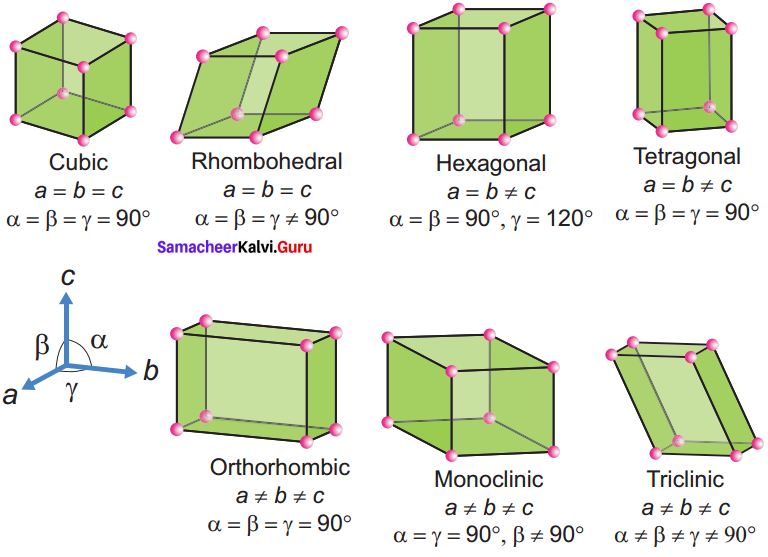1. Cubic – NaCl
2.  Tetragonal – TiO2
3. Orthorhombic – BaSO4
4. Hexagonal – ZnO
5. Monoclinic – PbCrO4
6. Triclinic – H3BO3
7. Rhombohedral – Cinnabar Cubic

They differ in the arrangements of their crystallographic axes and angles. Corresponding to the above seven, Bravis defined 14 possible crystal system as shown in the figure.

Question 6.
Distinguish between hexagonal close packing and cubic close packing.
Hexagonal close packing

1. aba arrangement
2. In this case, the spheres of the third layer are exactly aligned with those of the first layer
3. In HCP, tetrahedral voids of the second layer may be covered by the spheres of the third layer

Cubic close packing:

1. abc arrangement
2. In this case, the spheres of the third layer are not aligned with those of the first layer or second layer. Only when fourth layer is placed, its spheres are aligned with the first layer
3. In cep third layer may be placed above the second layer in a manner such that its sphere cover the octahedral voids

Question 7.
Distinguish tetrahedral and octahedral voids.
Tetrahedral void

1. A single triangular void in a crystal is surrounded by four (4) spheres and is called a tetrahedral void
2. A sphere of second layer is above the void of the first layer, a tetrahedral void is formed
3. This constitutes four spheres, three in the lower and one in upper layer. When the centres of these four spheres are joined a tetrahedron is formed
4. The radius of the sphere which can be accommodated in an octahedral hole without disturbing the structure should not exceed 0.414 times that of the structure forming sphere
5. Radius of an tetrahedral void $$\frac { r }{ R }$$ = 0.225

Octahedral void

1. A double triangular void like c is surrounded by six(6) spheres and is called an octahedral void
2. The voids in the first layer are partially covered by the spheres of layer now such a void is called a octahedral void
3. This constitutes six spheres, three in the lower layer and three in the upper layer. When the centers of these six spheres are joined an octahedron is formed
4. The sphere which can be placed in a tetrahedral hole without disturbing the close packed structure should not have a radius larger than 0.225 times the radius of the sphere forming the structure
5. Radius of a octahedral void $$\frac { r }{ R }$$ = 0.414

Question 8.
What are point defects?
If the deviation occurs due to missing atoms, displaced atoms or extra atoms the imperfection is named as a point defect. Such defects arise due to imperfect packing during the original crystallisation or they may arise from thermal vibrations of atoms at elevated temperatures.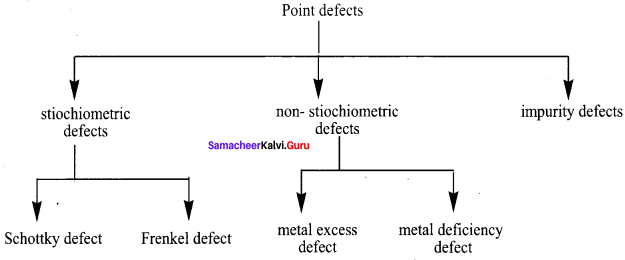Question 9.
Explain Schottky defect.
Schottky defect arises due to the missing of equal number of cations and anions from the crystal lattice. This effect does not change the stoichiometry of the crystal.Ionic solids in which the cation and anion are of almost of similar size show schottky defect.
Example: NaCl.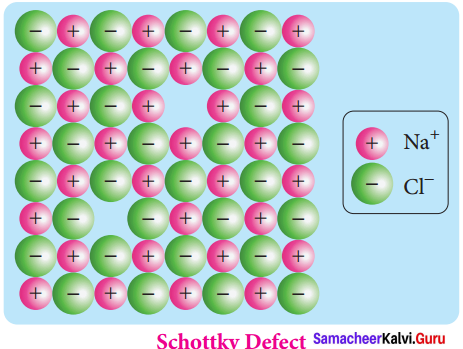Presence of large number of schottky defects in a crystal, lowers its density. For example, the theoretical density of vanadium monoxide (VO) calculated using the edge length of the unit cell is 6.5 g cm-3 but the actual experimental density is 5.6 gcm3. It indicates that there is approximately 14% Schottky defect in VO crystal. Presence of Schottky defect in the crystal provides a simple way by which atoms or ions can move within the crystal lattice.

Question 10.
Write short note on metal excess and metal deficiency defect with an example. Metal excess defect.
Metal excess defect arises due to the presence of more number of metal ions as compared to anions.Alkali metal halides NaCl, KCl show this type of defect.The electrical neutrality of the crystal can be maintained by the presence of anionic vacancies equal to the excess metal ions (or) by the presence of extra cation and electron present in interstitial position.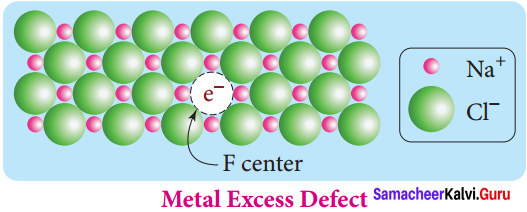For example, when NaCl crystals are heated in the presence of sodium vapour, Na+ ions are formed and are deposited on the surface of the crystal. Chloride ions (Cl) diffuse to the surface from the lattice point and combines with Na+ ion.

The electron lost by the sodium vapour diffuse into the crystal lattice and occupies the vacancy created by the Cl ions. Such anionic vacancies which are occupied by unpaired electrons are called F centers. Hence, the formula of NaCl which contains excess Na+ ions can be written as Na1+xCl.

Metal deficiency defect:
Metal deficiency defect arises due to the presence of less number of cations than the anions. This defect is observed in a crystal in which, the cations have variable oxidation states. For example, in FeO crystal, some of the Fe2+ ions are missing from the crystal lattice.

To maintain the electrical neutrality, twice the number of other Fe2+ ions in the crystal is oxidized to Fe3+ ions. In such cases, overall number of Fe2+ and Fe3+ ions is less than the O2- ions. It was experimentally found that the general formula of ferrous oxide is FexO, where x ranges from 0.93 to 0.98.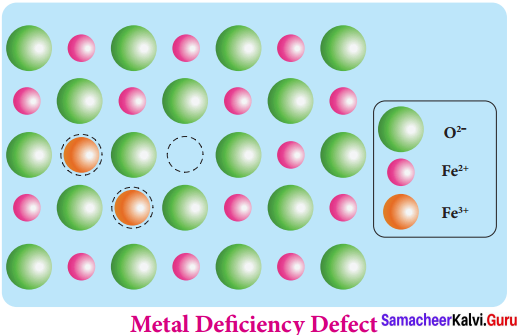Question 11.
Calculate the number of atoms in a fee unit cell.
Number of atoms in a fee unit cell,
= $$\frac { { N }_{ c } }{ 8 }$$ + $$\frac { { N }_{ f } }{ 2 }$$
= $$\frac { 8 }{ 8 }$$ + $$\frac { 6 }{ 2 }$$ = 1 + 3 = 4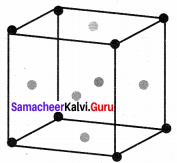Question 12.
Explain AAAA and ABABA and ABCABC type of three dimensional packing with the help of neat diagram.
1. AAAA type of three dimensional packing
This type of three dimensional packing arrangements can be obtained by repeating the AAAA type two dimensional arrangements in three dimensions, i.e., spheres in one layer sitting directly on the top of those in the previous layer so that all layers are identical.

All spheres of different layers of crystal are perfectly aligned horizontally and also vertically, so that any unit cell of such arrangement as simple cubic structure as shown in fig.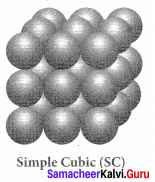In simple cubic packing, each sphere is in contact with 6 neighbouring spheres – Four in its own layer, one above and one below and hence the coordination number of the sphere in simple cubic arrangement is 6.

2. ABABA type of three dimensional packing:
In this arrangement, the spheres in the first layer (A type) are slightly separated and the second layer is formed by arranging the spheres in the depressions between the spheres in layer A as shown in figure.

The third layer is a repeat of the first. This pattern ABABAB is repeated throughout the crystal. In this arrangement, each sphere has a coordination number of 8, four neighbors in the layer above and four in the layer below.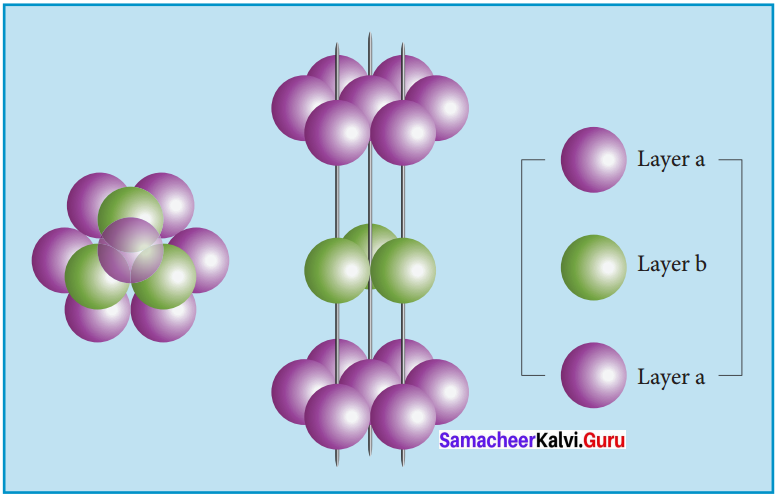3. ABCABC type of three dimensional packing:
In this arrangement (FCC) second layer spheres are arranged at the dips of first layer. Third layer spheres are arranged in a manner such that it cover the octahedral void. Then no longer third layer is similar to first or second layer.

Third layer gives different arrangement. Fourth layer spheres are similar to first layer. If the first, second and third layer are represented as A,B,C then this type of packing gives the arrangement of layers as ABCABC… (i.e.,), the first three layers do not resemble first, second and third layers respectively and the sequence is repeated.

with the addition of more layers. In this arrangement atoms occupy 74% of the available space and thus has 26% vacant space. The coordination number is 12. Voids – The empty spaces between the three dimensional layers are known as voids. There are two types of common voids possible. They are tetrahedral and octahedral voids.

Tetrahedral void – A void formed by three spheres of a layer in contact with each other and also with a sphere on the top or bottom layer is a hole between four spheres. The spheres are arranged at the vertices of a regular tetrahedron such a hole or void is called tetrahedral void.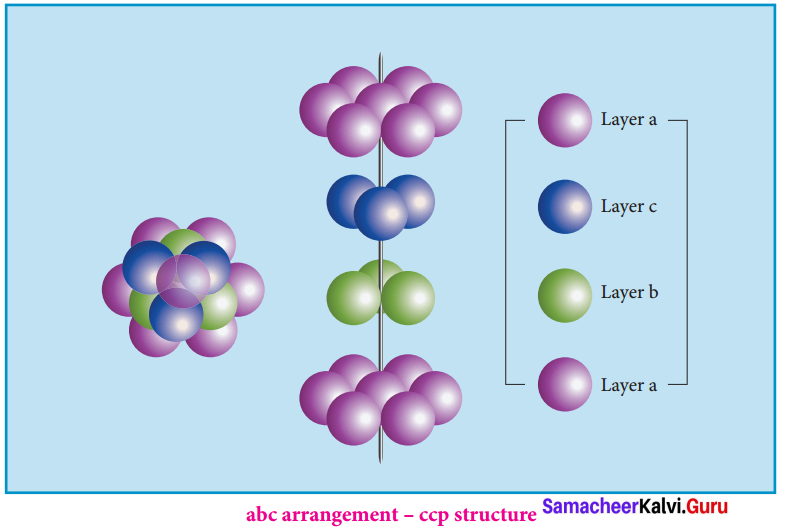Octahedral void:
A hole or void formed by three spheres of a hexagonal layer and another three spheres of the adjacent layer is a hole between six spheres. The spheres are arranged at the vertices of a regular octahedron. Such a hole or void is abc arrangement – ccp structure called octahedral void.

Question 13.
Why ionic crystals are hard and brittle?
The ionic compounds are very hard and brittle. In ionic compounds the ions are rigidly held in a lattice because the positive and negative ions are strongly attracted to each other and difficult to separate. But the brittleness of a compound is now easy to shift the position of atoms or ions in a lattice.

If we apply a pressure on the ionic compounds the layers shifts slightly. The same charged ions in the lattice comes closer. A repulsive forces arises between ‘ same charged ions, due to this repulsions the lattice structure breaks down chemical bonding.

Question 14.
Calculate the percentage efficiency of packing in case of body centered cubic crystal. Packing efficiency.
In body centered cubic arrangement the spheres are touching along the leading diagonal of the cube as shown in the In ∆ABC,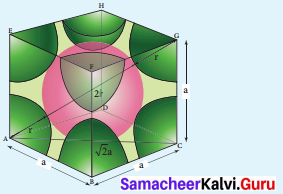AC2 = AB2 + BC2
AC = $$g\sqrt { { AB }^{ 2 }+{ BC }^{ 2 } }$$
AC = $$g\sqrt { { a }^{ 2 }+{ a }^{ 2 } }$$ = a2
In ∆ACG,
AG2 = AC2 + CG2
AG =$$g\sqrt { { AC }^{ 2 }+{ CG }^{ 2 } }$$
AG = $$\sqrt { { \left( \sqrt { 2 } a \right) }^{ 2 }+{ a }^{ 2 } }$$
AG = $$g\sqrt { { 2a }^{ 2 }+a^{ 2 } }$$ = $$g\sqrt { 3a^{ 2 } }$$ = $$\sqrt { 3 } a$$
i.e., $$g\sqrt { 3 }$$a = 4r
r = $$\frac { \sqrt { 3 } }{ 4 } a$$
∴ Volume of the sphere with radius ‘r’
= $$\frac { 4 }{ 3 }$$ πr3
= $$\frac { 4 }{ 3 }$$ π $$\left( \frac { \sqrt { 3 } }{ 4 } a \right)$$
= $$\frac { \sqrt { 3 } }{ 6 }$$ πa3
Number of spheres belong to a unit cell in bee arrangement is equal to two and hence the total volume of all spheres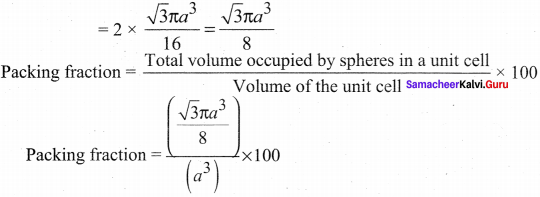i.e., 68% of the available volume is occupied.The available space is used more efficiently than in simple cubic packing

Question 15.
What is the two dimensional coordination number of a molecule in square close packed layer?
Square close packing – When the spheres of the second row are placed exactly above those of the first row. This way the spheres are aligned horizontally as well as vertically. The arrangement is AAA type. Coordination number is 4.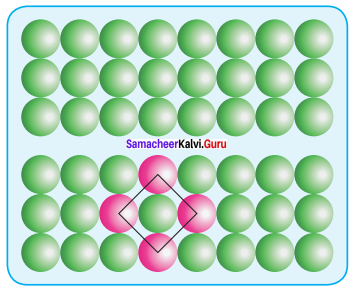Question 16.
Experiment shows that Nickel oxide has the formula Ni0.96 O1.00. What fraction of Nickel exists as of Ni2+ and Ni3+ ions ?
Let the number of Ni2+. Then the number of Ni3+ ion will be = (0.96 – x). Total number of cation, = 2 x + 3 (0.96 – x)
= 2x + 2.88 – 3x
= ( – x) + 2.88
Number of anions O2- ( – 2) x 1 = -2. Number of cations = Number of anions
– x + 2.88 2
– x = – 2.88 + 2
x =0.88
%of Ni as Ni2 =$$\frac { 0.08 }{ 0.96 } \times 100$$ = 91.66%
Number of Ni3+ ion will be = 0.96 – x
= 0.96 – 0.88 = 0.08
% of Ni as Ni3+ = $$\frac { 0.08 }{ 0.96 } \times 100$$ = 8.3 %

Question 17.
What is meant by the term “coordination number”? What is the coordination number of atoms in a bcc structure?

1. Coordination number – The number of nearest neighbours that surrounding a particle in a crystal is called the coordination number of that particle.
2. Coordination number of atoms in a bcc structure is 8

Question 18.
An element has bcc structure with a cell edge of 288 pm. the density of the element is 7.2 gcm-3. How many atoms are present ¡n 208g of the element.
An elemeñt has bec structure with a cell edge of 288 pm. The density of the element is 7.2 gcm-3. For the Bec structure, n = 2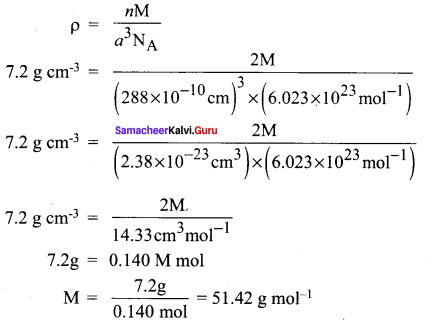By mole concept, 51.42 g of the element contains 6.023 x 1023 atom 208 g of the element will contain
= $$\frac { 6.023\times { 10 }^{ 23 }\times 208 }{ 51.42 }$$ atoms
= 24.17 x 1023 atoms (or) 2.417 x 1024 atoms

Question 19.
Aluminium crystallizes in a cubic close packed structure. Its metallic radius is 125pm. Calculate the edge length of unit cell.
Given, Radius (r) = 125 pm
Edge length of unit cell (a) = ?
Since aluminium crystallizes in Face centered cubic
r= $$\frac { a\sqrt { 2 } }{ 4 }$$ (or) r=$$\frac { a }{ 2\sqrt { 2 } }$$
a = r x 2 x $$g\sqrt { 2 }$$
= 125 x 2 x 1.414
a = 353.5 pm

Question 20.
If NaCI is doped with 102 mol percentage of strontium chloride, what is the concentration of cation vacancy?
We know that two Na+ ions are replaced by each of the Sr2+ ions while SrCl2, is doped with NaCI. But in this case, only one lattice point is occupied by each of the Sr2+ ions and produce one cation vacancy.

Here 10-2 mole of SrCI2, is doped with 100 moIes of NaCI. Thus, cation vacancies produced by NaCi = 10-2 mol. Since, 100 moles of NaCl produces cation vacancies after doping = 10-2 mol. Therefore, I mole of NaCl will produce cation vacancies after doping

= $$\frac { { 10 }^{ -2 } }{ 100 }$$= 10-4mol
:. Total cationic vacancies,
= 10 x 6.023 x 1023
= 6.023 x 1019 vacancies

Question 21.
KF crystallizes in fcc structure like sodium chloride, calculate the distance between K+ and F in KF. (given : density of KF is 2.48 g cm-3)
Density of KF 2.48 g cm-3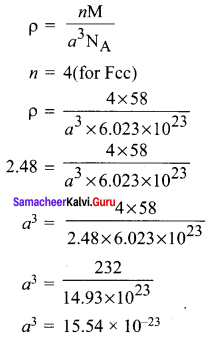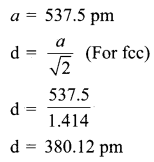Question 22.
An atom crystallizes ¡n fcc crystal lattice and has a density of 10 gcm3 with unit cell edge length of 100pm. calculate the number of atoms present in 1 g of crystal.
Given, Density = 10 g cm-3
mass = 1 g
Edge length of unit cell = 100 pm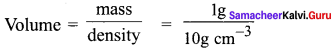= 0.1 cm3
Volume of unit cell = a3
= (100 x 10-10cm)3
= 1 x 10-24 cm3
Number of unit cell in 1 g of crystal,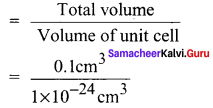The given unit cell is of FCC type. Therefore. it contains 4 atoms.
0.1 x 1024 unit cells will contain 4 x 0.1 x 1024 = 4 x 1023atoms

Question 23.
Atoms X and Y form bcc crystalline structure. Atom X is present at the corners of the cube and Y is at the centre of the cube. What is the formula of the compound?
Atoms X and Y form bcc crystalline structure. Atom X is present at the corners of the cube Atom Y is present at the centre of the cube.
No of atoms of X in the unit cell = $$\frac { { N }_{ c } }{ 8 }$$ = $$\frac { 8 }{ 8 }$$ = 1
No of atoms of Y in the unit cell = $$\frac { { N }_{ b } }{ 1 }$$ = $$\frac { 1 }{ 1 }$$ = 1
Ratio of atoms X : Y = 1 : 1
Hence formula of the compound = XY.

Question 24.
Sodium metal crystallizes in bcc structure with the edge length of the unit cell 4.3 x 10-8 cm. Calculate the radius of sodium atom.
Edge length of the unit cell (a) = 4.3 x 10-8cm
Radius of sodium atom (r) = ?
For bcc structure, r = $$\frac { \sqrt { 3 } }{ 4 } a$$
= $$\frac { \sqrt { 3 } }{ 4 } a$$ (4.3 x 10-8cm)
$$\frac { 1.732\times 4.3\times { 10 }^{ -8 } }{ 4 }$$
= $$\frac { 1.732 }{ 4 } \times { 10 }^{ -8 }cm$$
= 1.86 x 10-8 cm = l.86Å

Question 25.
Write a note on Frenkel defect.
Frenkel defect arises due to the dislocation of ions from its crystal lattice. The ion which is missing from the lattice point occupies an interstitial position. This defect is shown by ionic solids in which cation and anion differ in size.

Unlike Schottky defect, this defect does not affect the density of the crystal. For example AgBr, in this casc, small Ag ion leaves its normal site and occupies an interstitial position as shown in the figure.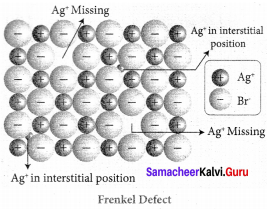Samacheer Kalvi 12th Chemistry Solid State Evaluate Yourself

Question 1.
An element has a face centered cubic unit cell with a length of 352.4 pm along an edge. The density of the element is 8.9 gcm-3. How many atoms are present in loo g of an element?
Mass = 100g
Density = 8.9 g cm-3
Edge length = 352.4 pm
(a) 352.4 x 10-10cm
Volume of the unit cell,
a3 = (352.4 x 1010cm) 34.37 x 1023 cm3
Volume of 100 g of an element.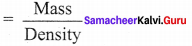Therefore number of unit cells,
$$\frac { 11.23 }{ 4.37\times { 10 }^{ -23 } }$$ = 11.23 = 2.56 x 1023
Since each Fcc cube contains 4 atoms, therefore total number of atoms in 100 g.
= 4 x (2.56 x 1023) = 10.24 x 1023 atoms

Question 2.
Determine the density of CsCl which crystallizes in a bcc type structure with an edge length 412.1 pm.
Molar mass of cscl = 168.5 g /mol
Number atoms present in per unit cell for bcc (cscl)
n = 1
Edge length (a) = 412.1 pm
Density (ρ) = ?
ρ = $$\frac { nM }{ { a }^{ 3 }{ N }_{ A } }$$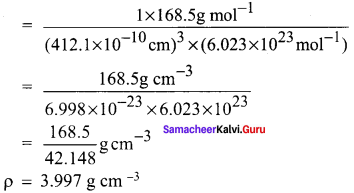Question 3.
A face centered cubic solid of an element (atomic mass 60) has a cube edge of 4 Å. Calculate its density.
For FCC unit cell n = 4
Edge length (a) = 4Å = 4 x 10-8cm
Mass (M) = 60 g mol-1
Density (p) = ?
ρ = $$\frac { nM }{ { a }^{ 3 }{ N }_{ A } }$$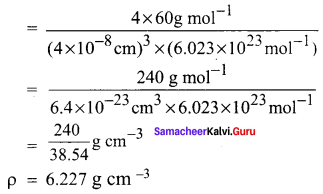### Samacheer Kalvi 12th Chemistry Solid State Additional Questions

Samacheer Kalvi 12th Chemistry Solid State 1 Mark Questions and Answers

Question 1.
Consider the following statements
(i) Solids have definite volume and shape
(ii) Solids are rigid and compressible
(iii) Solids have weak cohesive forces

Which of the above statemetns is / are not correct?
(a) (i) only
(b) (ii) & (iii) only
(c) (iii) only
(d) (i) & (ii) only
(b) (ii) & (iii) only

Question 2.
Which one of the following is an ionic crystal?
(a) Glass
(b) Rubber
(c) NaCl
(d) SiO2
(c) NaCl

Question 3.
Which one of the following is an amorphous solid?
(a) Glass
(b) SiO2
(c) NaCl
(d) Na
(a) Glass

Question 4.
Which one of the following is an example for molecular crystals?
(a) Diamond
(b) Silica
(c) Glass
(d) Naphthalene
(d) Naphthalene

Question 5.
Which one of the following is an example for atomic solids?
(a) Frozen elements of group 18
(b) Group 17 elements
(c) Inner – transition elements
(d) chalcogens
(a) Frozen elements of group 18

Question 6.
Which one of the following is a covalent crystal?
(a) Glass
(b) Diamond
(c) Anthracene
(d) Glucose
(b) Diamond

Questions 7.
Consider the following statements.
(i) Crystalline solids have irregular shape
(ii) Generally crystalline solids are anisotropic in nature
(iii) Heat of fusion of crystalline solids are not definite

Which of the above statements is / are correct?
(a) (i) & (iii)
(b) (i) only
(c) (iii) only
(d) (ii) only
(d) (ii) only

Question 8.
Consider the following statements.
(i) Amorphous solids are isotropic like liquids
(ii) Amorphous solids are considered as pseudo solids
(iii) Amorphous solids have sharp melting points

Which of the above statemetns is / are correct?
(a) (i) only
(b) (ii) only
(c) (i) & (ii)
(d) (i) & (iii)
(c) (i) & (ii)

Question 9.
In an ionic crystal, both cations and anions are bound together by ………..
(a) Strong electrostatic attractive forces
(b) Weak electrostatic attractive forces
(c) Vanderwaals forces of attraction
(d) Weak cohesive forces
(a) Strong electrostatic attractive forces

Question 10.
Molecular solids contains neutral molecules held together by ………..
(a) strong cohesive forces
(b) weak vanderwaals forces
(c) weak ionic forces
(d) strong electrostatic forces
(b) weak vanderwaals forces

Question 11.
Which is used inside pencils and in many lubricants?
(b) charcoal
(c) graphite
(d) coke
(c) graphite

Question 12.
In non polar molecular solids, molecules are held together by ………..
(a) London forces
(b) weak vanderwaals forces
(c) Strong electrostatic forces
(d) strong cohesive forces
(a) London forces

Question 13.
Which one of the following is non-polar molecular solids?
(a) Diamond
(b) SiC
(c) Anthracene
(d) Glass
(c) Anthracene

Question 14.
Silicon carbide is an example of ………..
(a) Ionic solid
(b) Covalent solid
(c) Polar molecular solid
(d) Non – polar molecular solid
(b) Covalent solid

Question 15.
Naphthalene is an example of ………..
(a) ionic solid
(b) covalent solid
(c) non polar molecular solid
(d) polar molecular solid
(c) non polar molecular solid

Question 16.
Solid NH3 solid CO2 are examples of ………..
(a) Covalent solids
(b) polar molecular solids
(c) molecular solids
(d) ionic solids
(b) polar molecular solids

Question 17.
Solids ice, glucose are examples of ………..
(a) metallic solids
(b) ionic solids
(c) hydrogen bonded molecular solids
(d) non polar molecular solids
(c) hydrogen bonded molecular solids

Question 18.
Consider the following statements.
(i) metallic solids possess high electrical and thermal conductivity
(ii) solid ice are soft solids under room temperature
(iii) In non polar molecular solids constituent molecules are held together by strong electrostatic forces of attraction

Which of the above statements is / are not correct?
(a) (i) & (ii)only
(b) (iii) only
(c) (ii) only
(d) (i) only
(b) (iii) only

Question 19.
Each atom in the comer of the cubic unit cell is shared by how many unit cells?
(a) 8
(b) 6
(c) 1
(d) 12
(a) 8

Question 20.
Which is the coordination number of each atom in a simple cubic unit cell?
(a) 8
(b) 6
(c) 12
(d) 4
(b) 6

Question 21.
The number of atoms belongs to fcc unit cell is ………..
(a) 2
(b) 4
(c) 6
(d) 12
(a) 2

Question 22.
The number of atoms in fee unit cell is ………..
(a) 2
(b) 4
(c) 6
(d) 8
(b) 4

Question 23.
The atoms the face centre is being shared by ………..
(a) 4
(b) 8
(c) 2
(d) 6
(c) 2

Question 24.
An atom present at the body centre be longs to only unit cell ………..
(a) 1
(b) 2
(c) 4
(d) 8
(a) 1

Question 25.
Which one of the following is known as Bragg’s equation’?
(a) d = $$\frac { 2sinθ }{ nλ }$$
(b) d = $$\frac { nλ }{ 2sinθ }$$
(c) d = $$\frac { d }{ sinθ }$$
(d) d = $$\frac { 2sinθ }{ nλ }$$
(b) d = $$\frac { nλ }{ 2sinθ }$$

Question 26.
Which one of the following formula is used to calculate the density of the unit cell ?
(a) ρ = $$\frac { nM }{ { a }^{ 3 }{ N }_{ A } }$$
(b) ρ = $$\frac { { a }^{ 3 }{ N }_{ A } }{ nM }$$
(c) ρ = $$\frac { { N }_{ A } }{ { a }^{ 3 }{ N }M }$$
(d) ρ = $$\frac { { a }^{ 3 }{ N }_{ A } }{ n }$$
(a) ρ = $$\frac { nM }{ { a }^{ 3 }{ N }_{ A } }$$

Question 27.
Which is the packing fraction in simple cubic unit cell?
(a) 52.31%
(b) 100%
(c) 68%
(d) 75%
(a) 52.3 1%

Question 28.
The packing fraction in bcc arrangement is ………..
(a) 52.3 1%
(b) 68%
(c) 100%
(d) 80%
(b) 68%

Question 29.
Which is the coordination number in both hep and ccp arrangements?
(a) 12
(b) 6
(c) 4
(d) 8
(a) 12

Question 30.
What is the coordination number of B2O3?
(a) 4
(b) 6
(c) 8
(d) 3
(d) 3

Question 31.
Which one of the following is the structure of B2O3 ?
(a) Tetra hedral
(b) Octahedral
(c) Trigonal planar
(d) Cubic
(c) Trigonal planar

Question 32.
The coordination number of zinc sulphide is ………..
(a) 3
(b) 4
(c) 6
(d) 8
(b) 4

Question 33.
The coordination number of CSCI ¡s ………..
(a) 3
(b) 4
(c) 6
(d) 8
(d) 8

Question 34.
Which one of the following is the coordination number of NaCl?
(a) 3
(b) 4
(c) 6
(d) 8
(c) 6

Question 35.
Which one of the following is the packing efficiency in fcc unit cell?
(a) 74%
(b) 52.6 1%
(c) 100%
(d) 68%
(a) 74%

Question 36.
Which one of the following is an example for schoriky defect?
(a) NaCI
(b) AgBr
(c) KCI
(d) FeS
(a) NaCl

Question 37.
Which one of the following is an example for Frenkel defect?
(a) NaCl
(b) AgCI
(c) AgBr
(d) AgNO3
(c) AgBr

Question 38.
Metal excess defect is possible in ………..
(a) AgCI
(b) AgBr
(c) KCl
(d) Fes
(c) KCl

Question 39.
Which one of the following is the metal deficiency defect?
(a) FeO
(b) ZnO
(c) KCl
(d) NaCl
(a) FeO

Question 40.
Which one of the following shows non- stoichiometric defect?
(a) FeO
(b) AgBr
(c) ZnO
(d) Both a and c
(d) Both a and c

II. Fill in the blanks

1. Naphthalene,Anthracene and glucose are examples of ……………
2. The best examples of covalent crystals are …………… and ……………
3. Frozen elements of group 18 are called ……………
4. Glass, Rubber, plastics are the examples of …………… solids.
5. …………… means uniformity in all directions.
6. Crystalline solids are …………… and they show different values of physical properties when measured along different directions.
7. NaCl and KCl are the examples of …………… crystals.
8. Diamond and silicon carbide are the examples of …………… solids.
9. In molecular solids, the neutral molecules are held together by weak ……………
10. …………… is a component of many lubricants for example cycle chain oil.
11. In non polar molecular solids constituent molecules are held together by ……………
12. In solids CO2 solid NH3 the molecules are held together by strong ……………
13. Glucose and urea are generally …………… under room temperature.
14. …………… solids possess excellent electrical and thermal conductivity.
15. The regular arrangement of the ions throughout the crystal is called a ……………
16. The basic repeating structural unit of a crystalline solid is called a ……………
17. The number of the nearest neighbours that surrounding a particle in a crystal is called the ……………
18. A unit cell that contains only one lattice point is called a ……………
19. There are …………… primitive crystal systems.
20. The coordination number of bcc is ……………
21. Each atom in the comer of cubic unit cell is shared by …………… neighbouring unit cells.
22. The number of atoms in bcc unit cell is ……………
23. The number of atoms in a fee unit cell is ……………
24. …………… is the most powerful tool for the determinaiton of crystal structure.
25. Only …………… of the available volume is occupied by the spheres in simple cubic packing
26. Of all the metals in the periodic table, only …………… crystallizes in simple cubic pattern.
27. In bcc cubic pattern …………… of the available volume is occupied.
28. If the third layer arrangement is aba arrangement, it is called …………… arrangement.
29. If third layer arrangement is abc arrangment, it is known as …………… arrangement.
30. In both …………… and …………… arrangements, the coordination number of each sphere is 12.
31. The packing efficiency of fee unit cell is ……………
32. …………… defect arises due to the missing of equal number of cations and anions from the crystal lattice.
33. Presence of large number of schottky defects in a crystal, lowers its ……………
34. Vanadium monoxide shows …………… defect.
35. …………… arises due to the dislocation of ions from its crystal lattice.
36. …………… arises due to the presence of more number of metalions as compared to anions.
37. Zno is …………… at room temperature but when it is heated it becomes in colour.
38. …………… arises due to the presence of less number of cations than the anions.
39. …………… is the appearance of an electrical potential across the sides of the crystal, when it is subjected to mechanical stress.
40. Stoichiometric defects in an ionic solid is also called …………… or …………… defect.

1. Molecular crystals
2. Diamond, SiO2
3. Atomic solids
4. Amorphous
5. Isotropy
6. Anisotropic
7. Ioni
8. Covalent
9. Vander waals forces
10. Graphite
11. London forces
12. dipole-dipole interactions
13. Soft solids
14. Metallic
15. Crystal lattice
16. Unit cell
17. Coordination number
18. Primitive unit cell
19. Seven
20. Eight
21. Eight
22. Two
23. Four
24. X-ray diffraction analysis
25. 52.31%
26. Polonium
27. 68%
28. hep or hexagonal close packed
29. ccp or cubic close packed
30. hep, ccp
31. 74%
32. Schottky
33. density
34. Schottky defect
35. Frenkel defect
36. metal excess defect
37. Colourless, yellow
38. Metal deficiency
39. Piezoelectricity
40. intrinsic or thermodynamic

III. Match the following:

Question 1.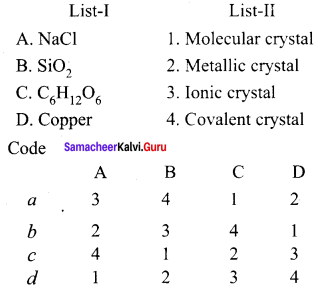(a) 3, 4, 1, 2

Question 2.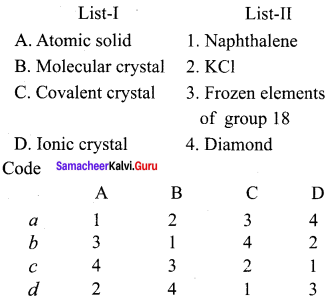(b) 3, 1, 4, 2

Question 3.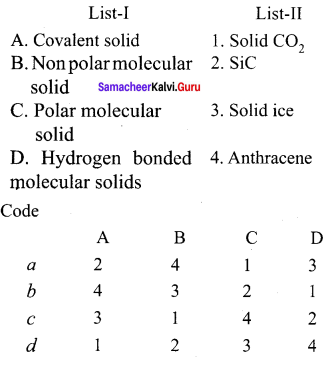(a) 2, 4, 1, 3

Question 4.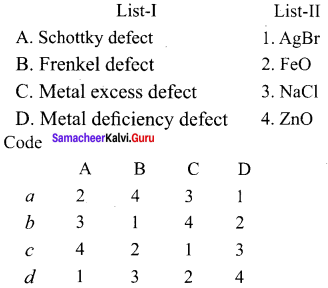(b) 3, 1, 4, 2

Question 5.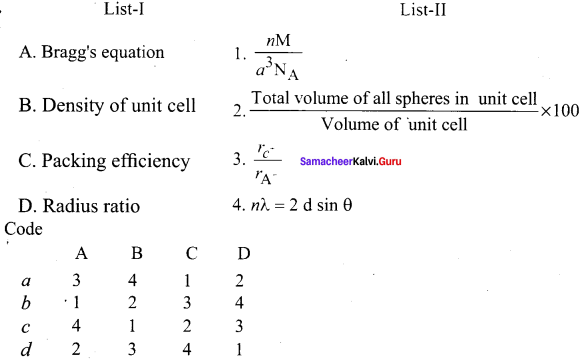(c) 4, 1, 2, 3

IV. Assertion and Reason

Question 1.
Assertion (A): Amorphous solids are isotropic in nature.
Reason (R): In amorphous solids, they have identical values of physical properties such as refractive index, electrical conductance in all directions which is called isotropy.
(a) Both A and R are correct and R is the correct explanation of A.
(b) Both A and R are correct but R is not the correct explanation of A
(c) A is correct but R is wrong
(d) A is wrong but R is correct
(a)Both A and R are correct and R is the correct explanation of A.

Question 2.
Assertion (A): Crystalline solids are anisotropic in nature.
Reason (R): Anisotropy is the property which depends on the direction of measurement. Crystalline solids are anisotropic and they show different values of physical properties when measured along different directions.
(a) Both A and R are correct and R is the correct explanation of A.
(b) Both A and R are correct but R is not the correct explanation of A
(c) A is correct but R is wrong
(d) A is wrong but R is correct
(a)Both A and R are correct and R is the correct explanation of A.

Question 3.
Assertion (A): Ionic solids do not conduct electricity in solid state but in molten state they conduct electricity.
Reason (R): In solid state, the ions are fixed in their lattice positions but in molten state, the ions are free to move and conduct electricity.
(a) Both A and R are correct but R is not correct explanation of A.
(b) Both A and R are correct and R is the correct explanation of A
(c) A is correct but R is wrong
(d) A is wrong but R is correct
(A)Both A and R are correct and R is the correct explanation of A

Question 4.
Assertion (A): Diamond and Silicon carbide are very hard and have high melting point.
Reason (R): In covalent solids, the atoms are bound together in a three dimensional network entirely by covalent bonds.
(a) Both A and R are correct and R is the correct explanation of A.
(b) Both A and R are correct but R is not the correct explanation of A
(c) A is correct but R is wrong
(d) A is wrong but R is correct
(a)Both A and R are correct and R is the correct explanation of A.

Question 5.
Assertion (A): Solid CO2 Solid NH3 have higher melting points.
Reason (R): The constituents are molecules formed by polar covalent bonds. They are held together by relatively strong dipole- dipole interactions.
(a) A is correct but R is wrong.
(b) A is wrong but R is correct
(c) A and R are correct and R is the correct explanation of A
(d) A and R are correct but R is not the correct explanation of A
(c) A and R are correct and R is the correct explanation of A

Question 6.
Assertion (A): Solid ice, Glucose are generally soft solids under room temperature.
Reason (R): The constituents are held together by strong electrostatic forces of attraction.
(a) Both A and R are correct and R is the correct explanation of A
(b) Both A and R are correct and R is not the correct explanation of A
(c) A is correct but R is wrong.
(d) A is wrong but R is correct
(c) A is correct but R is wrong.

Question 7.
Assertion (A): In bcc, the available volume is more efficiently used than in simple cubic packing.
Reason (R): In simple cubic arrangement, the number of spheres belongs to a unit cell is equal to one whereas in bcc, it is equal to 2.
(a) Both A and R are correct and R is the correct explanation of A
(b) Both A and R are correct R is not the correct explanation of A
(c) A is correct but R is wrong.
(d) A is wrong but R is correct
(a) Both A and R are correct and R is the correct explanation of A

Question 8.
Assertion (A): B2 O3 has trigonal planar structure.
Reason (R): The ratio of radius of cation and anion $$\frac { { r }_{ { c }^{ + } } }{ { r }_{ { A }^{ – } } }$$ = 0.155 – 0.225 plays an important role in determining the structure . and B2 O3 has coordination number as 3 and has trigonal planar structure.
(a) Both A and R are correct and R is the correct explanation of A
(b) Both A and R are correct but R is not the correct explanation of A
(c) A is correct but R is wrong.
(d) A is wrong but R is correct
(a) Both A and R are correct and R is the correct explanation of A

Question 9.
Assertion (A): Schottky defect does not change the stoichiometry of the crystal.
Reason (R): This defect arises due to the missing of equal number of cations and anions from the crystal lattice.
(a) Both A and R are correct but R is not the correct explanation of A
(b) Both A and R are correct and R is the correct explanation of A
(c) A is correct but R is wrong.
(d) A is wrong but R is correct
(a) Both A and R are correct and R is the correct explanation of A

Question 10.
Assertion (A): Zinc oxide is colourless at room temperature but on heating it becomes yellow in colour.
Reason (R): On heating Zinc loses oxygen and thereby forming free zn2+ ions. The excess zn2+ ions move to interstitial sites and the electrons also occupy interstitial positions.
(a) Both A and R are correct and R is the correct explanation of A
(b) Both A and R are correct R is not the correct explanation of A
(c) A is correct but R is wrong.
(d) A is wrong but R is correct
(a) Both A and R are correct and R is the correct explanation of A

V. Find the odd one out

Question 1.
(a) Plastic
(b) Rubber
(c) Glucose
(d) Glass
(c) Glucose It is a molecular solid where as others are amorphous solids.

Question 2.
(a) Anthracene
(b) Naphthalene
(c) Glucose
(d) Sodium chloride
(d) Sodium chloride It is ionic crystall where as others are molecular crystals.

Question 3.
(a) Sodium
(b) Pottasium
(c) Frozen elements of group 18
(d) Gold
(c) Frozen elements of group 18 It is atomic solid where as others are metallic solids.

Question 4.
(a) Solid CO2
(b) Solid ice
(c) Glucose
(d) Urea
(a) Solid CO2 It is a polar molecular solid where as others are hydrogen bonded molecular solids.

Question 5
(a) Cubic
(b) Rhombohedral
(c) Hexagonal
(d) Cyclic
(d) Cyclic It is a ring structure where as other primitive crystal systems.

VI. Find the odd correct pair

Question 1.
(a) Glass, plastic
(b) Rubber, ice
(c) Nacl, Glucose
(d) Urea, solid NH3
(a) Glass, plastic (Amorphous solids)

Question 2.
(a) NaCI, KCI
(b) FeO, ZnO
(e) AgBr, AgNO3
(d) VO, ZnO
(a) Naci, Kcl (schottky defect)

Question 3.
(a) Solid CO2 Solid ice
(b) Solid CO2 Solid NH3
(c) Graphite, Silicon carbide
(d) Naphthalene, Phenol
(b) Solid CO2 Solid NH3 Polar molecular solids

Question 4.
(a) NaCI, SiC
(b) Naphthalene, anthracene
(c) Solid ice, graphite
(d) Copper, KO
(b) Naphthalcne, anthracenc – It is non-polar molecular solid examples.

VII. Find out the odd incorrect pair

Question 1.
(a) NaCl, KCl
(b) Naphthalenc, anthracene
(c) Solid CO2, Solid NH3
(d) Diamond, solid ice
(d)Diamond, solid ice

Question 2.
(a) Cu, Fc
(b) Glucose, Urca
(c) Diamond, SiC
(d) Benzene, glucose
(d) Benzene, glucose

Samacheer Kalvi 12th Chemistry Solid State 2 Mark Question and Answers

Question 1.
What are crystalline solid? Give example.
A crystalline solid is one in which its constituents (atoms, ions or molecules) have an orderly arrangement extending over a long range which has three dimensional pattern. Example – NaCl.

Question 2.
What are amorphous solid? Give example.
In an amorphous solid, the constituents are randomly arranged. It is a short range arrangement of constituents. Eg – Glass.

Question 3.
What are covalent solids? Give example.
In covalent solids, the constituents (atoms) are bound together in a three dimensional network entirely by covalent bonds. Examples. Diamond, Silicon carbide.

Question 4.
Silicon carbide is very hard. Justify this statement.
Silicon carbide is very hard. It is a covalent solid contains the atoms which are bound together in a three dimensional network entirely by covalent bonds. So the covalent network crystal SiC is very hard and have high melting point.

Question 5.
Write a note about molecular solids.

1. In molecular solids, the constituents are neutral molecules. They are held together by weak vander waals forces.
2. Molecular solids are soft and they do not conduct electricity. Eg – Solid CO2

Question 6.
What are non-polar molecular solids? Give example.

1. In non polar molecular solids, constituent molecules are held together by weak dispersion forces or London forces.
2. They have low melting points and are usually in liquids or gaseous state at room temperature. Eg., Naphthalene, anthrancene.

Question 7.
What are hydrogen bonded molecular solids? Give example.

1. Molecular solids in which the constituents are held together by hydrogen bonds.
2. They are generally soft solids under room temperature. Examples., Solid ice, Glucose, Urea.

Question 8.
Define crystal lattice.
The regular arrangement of these species throughout the crystal is called a crystal lattice.

Question 9.
Define coordination number.

1. A crystal may be considered to consist of large number of unit cells, each one in direct contact with its nearer neighbour and all similarly oriented in space.
2. The number of the nearest neighbours that surrounding a particle in a crystal is called coordination number of that particle.

Question 10.
Draw the tetragonal crystal systems.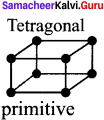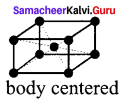Question 11.
Draw the hexagonal primitive crystal structure.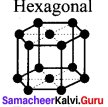Question 12.
Draw the typec of monoclinic primitive cubic crystals.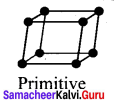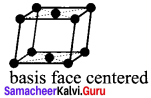Question 13.
Draw the primitive cubes.

1. trigonal
2. triclinic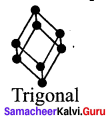Question 14.
What is Bragg’s equation?
1. X-ray diffraction analysis is the most powerful tool fiw the determination of crystal structure.

2. The interplanar distance (d) between two successive planes of atoms can be calcuLated using the following equation form the X-ray diffraction data 2d sin θ = nλ. The equation is known as Bragg’s equation.

Where λ = wavelength of X-ray – d = Interplanar distance, θ The angle of diffraction – n = order of reflection. By knowing the values of θ, λ and n. we can calculate the value of d. d = $$\frac { nλ }{ 2Sinθ }$$ Using these values, the edge of the unit cell can be calculated.

Question 15.
What is meant by linear arrangement of spheres in one direction?
1. In a specific direction, there is only one possibility to arrange the spheres in one direction.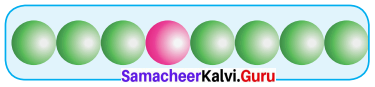2. In this arrangement, each sphere is in contact with two neighbouring spheres on either.

Question 16.
What is meant by piezo electricity?
Piezo electricity is the appearance of an electrical potential across the sides of a crystal. When you subject it to mechanical stress. The word piezo electricity means electricity resulting from pressure and latent heat. Even the inverse is possible which is known as inverse piezo electric effect.

Question 17.
Why are solids rigid?
In a solid, the constituent particles are very closely – packed. Hence, the forces of attraction among these particles are very strong, that is why they are rigid.

Question 18.
Why do solids have a definite volume?
The constituent particles of a solid have fixed positions and are not free to move i.e., they possess rigidity. That is why they have a definite volume.

Question 19.
Classify the following as amorphous or crystalline solids: Polyurethane, naphthalene, benzoic acid, teflon, potassium nitrate, cellophane, polyvinyl chloride, fibre glass, copper.
Amorphous solids: Polyurethane, teflon, cellophane, polyvinyl chloride, fibre glass. Crystalline solids: Benzoic acid, naphthalene, potassium nitrate, copper.

Question 20.
Why is glass considered as super cooled liquid?
Glass is an amorphous solid. Like liquids it has a tendency to flow, though very slowly. The proof of this fact is that glass panes in the windows or doors of old buildings are invariably found to be slightly thicker at the bottom than at the top.

Question 21.
Refractive index of a solid is observed to have the same value along all directions. Comment on the nature of this solid. Would it show cleavage property?
As the solid has same value of refractive index along all directions, this means that it is isotropic and hence amorphous. Being an amorphous solid, it would not show a clean cleavage when cut with a knife. Instead it would break into pieces with irregular surfaces.

Question 22.
Classify the following solids in different categories based on the nature of intermolecular forces operating in them: Potassium sulphate, tin, benzene, urea, ammonia, water, zinc sulphide, graphite, rubidium, argon, silicon carbide.

1. Potassium sulphate – Ionic
2. Tin – Metallic
3. Benzene – Molecular (non polar)
4. Urea – Molecular (polar)
5. Ammonia – Molecular (hydrogen bonded)
6. Water – Molecular (hydrogen bonded)
7. Zinc Suiphide – Ionic
8. Graphite – Covalent or Network
9. Rubidium – Metallic
10. Argon – Molecular (non polar)
11. Silicon carbide – Covalent or Network

Question 23.
Solid A is a very hard electrical insulator in solid as well as in molten state, and melts at extremely high temperature. What type of solid is it?
Covalent or Network solid like SiO2 (quartz) or SIC or C (diamond).

Question 24.
Ionic solids conduct electricity in molten state but not in solid state. Explain.
In the molten state, ionic solids dissociate to give free ions and hence can conduct electricity. However in the solid state, as the ions are not free to move but remain held together by strong electrostatic forces of attraction, so they cannot conduct electricity in the solid state.

Question 25.
An element with molar mass 2.7 x 10-2 kg mol-1 forms a cubic unit cell with edge length 405 pm. If the density is 2.7 x 103 kg m-3, what ¡s the nature of the cubic unit cell?
DensityThus, there are 4 atoms of elements present per unit cell, hence, the cubic unit cell must be facecentred or cubic close – packed (cep).

Question 26.
Ionic solids, which have anionic vacancies due to metal excess defect develop colour. Explain with the help of suitable example.
Taking the example of NaCl, when its crystals arc heated in presence of sodium vapour some chloride ion leave their lattice sites to combine with sodium to form NaCI. For this released diffuses into the crystal to occupy the anion vacancies created by Cl ions.

The crystal now has excess of sodium. The sites occupied by unpaired electrons are called F – centres. They impart yellow colour to the crystal because they absorb energy from the visible light and get excited.

Question 27.
Classify each of the following solids as ionic, metallic, molecular, network (covalent) or amorphous.

1. Tetra phosphorous decoxide (P4 O10)
2. Ammonium Phosphate (NH4)3PO4
3. SIC
4. I2
5. P4
6. Plastic
7. Graphite
8. Brass
9. Rb
10. LiBr
11. Si

1. Tetra phosphorous decoxide (P4 O10) = Molecular solid
2. Ammonium Phosphate (NH4)3PO4 = Ionic solid
3. SiC = Covalent solid
4. I2 = Molecular solid
5. P4 = Molecular solid
6. Plastic Amorphous solid
7. Graphite = Covalent solid
8. Brass = Metallic solid
9. Rb = Metallic solid
10. LiBr = Ionic solid
11. Si = Covalent solid

Question 28.
What ¡s the formula of a compound in which the element Y forms ccp Lattice and atoms of X occupy 2/3rd of tetrahedral voids?
Number of element Y = n, Number of element X = 2 n x $$\frac { 2 }{ 3 }$$. As number of tetrahedral void = 2n
X : Y= $$\frac { 4n }{ 3 }$$ : n
Formula = X4 Y3

Question 29.
The energy required to vapourise one mole of copper is smaller than that of energy required to vapourise I mol of diamond. Why?
Copper is a metallic solid having metal-metal bonds while diamond is a covalent solid having covalent bonds.Metallic bonds are weaker than covalent bonds and thus less amount of energy is required to break metallic bonds than covalent bonds.

Question 30.
Assign reason for the following:

1. phosphorous doped silicon is a semiconductor.
2. Schottky defect lowers the density of a solid.

1. It is because its conductance is intermediate between conductor and insulator.
2. In Schottky defect, both cations and anions are missing which leads to lowering the density of a solid.

Samacheer Kalvi 12th Chemistry Solid State 3 Mark Questions and Answers

Question 1.
Distinguish between isotropy and anisotropy?
Isotropy:

1. Isotropy means uniformity in all directions.
2. Isotropy means having identical values of physical properties such as refractive index, electrical conductance in all directions.
3. Isotropy is the property of amorphous solids.

Anisotropy:

1. Anisotropy means non-uniformity in all directions.
2. Anisotropy is the property which depends on the direction of measurement. They show different values of physical properties when measured along different directions.
3. Anisotropy is the properly of crystalline solids.

Question 2.
What are polar molecular solids? Give example.

1. The constituents are molecules formed by polar covalent bonds.
2. They are held together by relatively strong dipole-dipole interactions.
3. They have higher melting points than the non-polar molecular solids. Eg., Solid CO2, Solid NH3.

Question 3.
Write a note about metallic solids.

1. In metallic solids, the lattice points are occupied by positive metal ions and a cloud of electrons pervades the space.
2. They are hard and have high melting point.
3. Metallic solids possess excellent electrical and thermal conductivity. They possess bright lustre.
4. Examples – Metals and metal alloys Cu, Fe, Zn, Ag, Ay, Cu – Zn etc.

Question 4.
What are characteristic parameters of a unit cell?
1. A basic repeating structural unit of a crystalline solid is called a unit cell.

2. A unit cell is characterised by the three edge lengths or lattice constants a,b and c and the angle between the edges a,P and y.

3.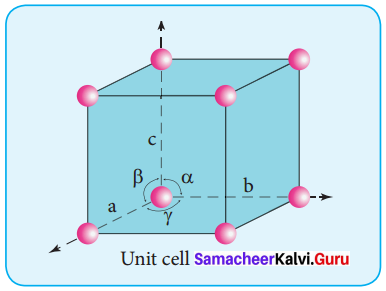Question 5.
What are type of unit cells? Give their names.

1. There are two types of unit cells, a) primitive b) Non – primitive.
2. A unit cell that contains only one lattice point is called a primitive unit cell, which is made up from the lattice points at each of the comers.
3. In the case of non-primitive unit cells, there are additional lattice points, either on a face of the unit cell or with in the unit cell.

Question 6.
Draw the seven types of primitive crystal systems.Question 7.
Draw the types of cubic crystal systems.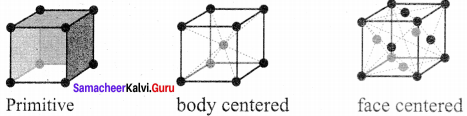Question 8.
Draw the types of orthorhombic cubic crystal systems.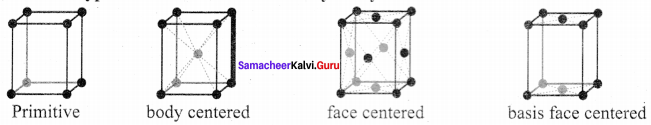Question 9.
Calculate the number of atoms belong to one unit cell of simple cubic unit cell(sc).
1. In the simple cubic unit cell, each corner is occupied by an identical atoms (or) ions (or) molecules and they touch along the edges of cube,do not touch diagonally. The coordination number of each atom is 6.

2. Each atom in the comer of the cubic unit cell is shared by 8 neighbouring unit cells and therefore atoms per unit cell is equal to $$\frac { { { N }_{ c } } }{ 8 }$$. where Nc is the number of atoms at the corners.

3. no of atoms in a Sc unit cell = $$\left( \frac { { N }_{ c } }{ 8 } \right)$$ = $$\left( \frac { 8 }{ 8 } \right)$$ = 1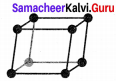Question 10.
Calculate the number of atoms per unit cell of bec type.
1. In a body centered cubic unit cell, each corner is occupied by an identical particle and in addition to that one atom occupied the body centre.

2. Those atoms which occupy the corners do not touch each other, however they all touch the one that occupies the body centre.

3. Hence each atom is surrounded by eight nearest neighbours and coordination number is 8. An atom present at the body centre belongs to only a particular unit cell i.e., unshared by other cell.
∴number of atoms in a bcc unit cell = $$\frac { { N }_{ c } }{ 8 }$$ + $$\frac { { N }_{ b } }{ 1 }$$ + $$\frac { 8 }{ 8 }$$ = $$\frac { 1 }{ 1 }$$ = 1 + 1 = 2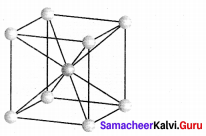Question 11.
How will derive the formula of density of a unit cell?
1. Using the edge length of a unit cell, we can calculate the density (p )of the crystal by considering a cubic unit cell as follows.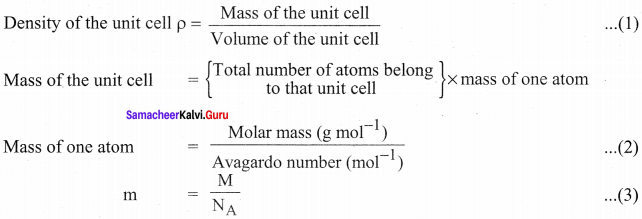Substitute the value (3) in (2)
Mass of the unit cell = n x $$\frac { M }{ { N }_{ A } }$$
For a cubic unit cell, all the edge lengths are equal. i.e., a = b = c
Volume of the unit cell = a x a x a = a3
∴Density of the unit cell = ρ = $$\frac { nM }{ { a }^{ 3 }{ N }_{ A } }$$

Question 12.
Calculate the packing fraction of siniple cubic arrangement
In a simple cubic arrangement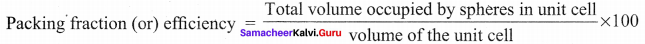Consider the cube with an edge length ‘a’
Volume of the cube with edge length a is = a x a x a = a3 …………….(l)
Let ‘r’ ¡s the radius of the sphere
From the figure a = 2 r ⇒ r = $$\frac { a }{ 2 }$$
:. volume of the sphere with radius r = $$\frac { 4 }{ 3 }$$ πr3
= $$\frac { 4 }{ 3 }$$ π $${ \left( \frac { a }{ 2 } \right) }^{ 3 }$$
= $$\frac { 4 }{ 3 }$$ π $$\left( \frac { { a }^{ 3 } }{ 8 } \right)$$
= $$\frac { { πa }^{ 3 } }{ 6 }$$ ……………………….(2)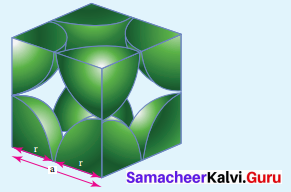In a simple cubic arrangement. number of spheres belongs to a unit cell equal to one.
∴Total volume occupied by the spheres in sc unit cell = 1 x $$\left( \frac { { πa }^{ 3 } }{ 6 } \right)$$ ……(3)
Dividing 3 by 1

Packing fraction = $$\left( \frac { \frac { \pi { a }^{ 3 } }{ 6 } }{ { a }^{ 3 } } \right) \times 100$$ = $$\frac { 100π }{ 6 }$$ = 52.3 1% Only 52.31% of the available volume is occupied by the spheres in simple cubic packing, making in efficient use of available space and hence minimizing the attractive forces.

Question 13.
What is meant by packing efficiency? How is ¡t measured?
1. There is some free space between the spheres of a single layer and the spheres of successive layers.

2. The percentage of total volume occupied by these constituent spheres gives the packing efficiency of an arrangement. For eg., in simple cubic arrangement.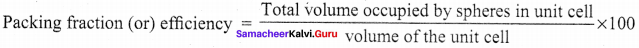Question 14.
Calculate the packing efficiency in fcc unit cell?
Total number of spheres belongs to a single fcc unit cell is 4. Volume of the sphere with radius r is = $$\frac { n }{ 2 }$$ π $${ \left( \frac { \sqrt { 2 } a }{ 4 } \right) }^{ 3 }$$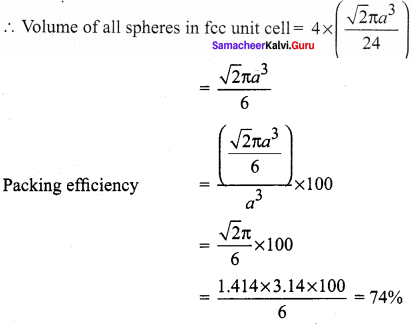Question 15.
How is radius ratio ¡s useful in determination of structure of an Ionic compound?

1. The structure of an ionic compound depends upon the stoichiometry and the size of the ions.
2. Generally in ionic crystals, the bigger anions are present in the close packed arrangements the cations occupy the voids.
3. The ratio of radius of cation and anion plays an important role in determining the structure.
4. For Eg;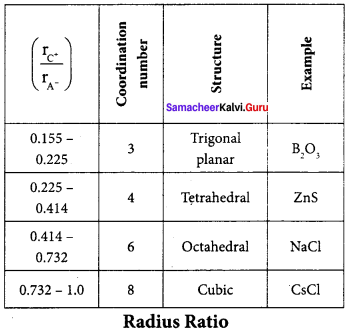Question 16.
What Is meant by impurity defect? Explain with example?

1. A method of introducing defects in ionic solids by adding impurity ions.
2. If the impurity ions are in different valence state from that of host, vaccancies are creited in the crystal lattice of the host.
3. For cg., addition of CdCl2 to silver chloride yields solid solutions where the divalent cation Cd2+ occupies the position of Ag2+
4. This will disturb the electrical neutrality of the crystal. In order to maintain the same, proportional number of Ag ions leave the lattice. This produces a cation vaccancy in the lattice, such kind of crystal defects are called impurity defects.

Question 17.
A compound is formed by two elements M and N. The element N forms ccp and atoms of M occupy 1/3 rd of the tetrahedral voids. What is the formula of the compound?
Suppose the atoms N in the ccp = n
∴ No. of tetrahedral voids = 2n
As 1/3 rd of the tetrahedral voids are occupied by atoms M, therefore,
No. of atoms M = $$\frac { 2n }{ 3 }$$
∴ Ratio of M : N = $$\frac { 2n }{ 3 }$$ : n = 2 : 3
Hence, the formula is M2N3

Question 18.
How many lattice points are there in one unit cell of each of the following lattice?

1. Face – centred cubic
2. Face – centred tetragonal
3. Body – centered

1. Lattice points in face-centred cubic lattice = 8 (at corners) + 6 (at the face centre) = 14
2. Face centred tetragonal = 8 (at corners) + 6 (at the face centre) = 14
3. Lattice points in body-centred cube = 8 (at corners) +1 (at the body centre) = 9

Question 19.
Explain:

1. The basis of similarities and differences between metallic and ionic crystals.
2. Ionic solids are hard and brittle.

1. Similarities:

• Both ionic and metallic crystals have electrostatic forces of attractiàn.
• In ionic crystals these forces are between oppositely charged ions. In metals,these forces are among the valence electrons and posityely charged kernels.
• Both have high melting point.

Differences:

• Ionic bond is strong due to electrostatic forces of attraction whereas metallic bond may be weak or strong depending upon the number of valence electrons and the size of kernels.
• In ionic bond, ions are not free to move. Hence, they cannot conduct electricity in solid state. They can do so only in molten state or in aqueous solution. ¡n metals, electrons are free to move. Hence, they conduct electricity in solid state.

2. Ionic crystals are hard due to strong electrostatic forces between them. They are brittle because ionic bond is non – directional.

Question 20.
ZnO is colourless at room temperature, while yellow when hot, why?
ZnO is colourless at room temperature. When it is heated, it becomes yellow in colour. On heating, it loses oxygen and thereby forming free Zn2+ ions. The excess Zn2+ ions move to interstitial sites and the electrons also occupy the interstitial positions.

Samacheer Kalvi 12th Chemistry Solid State 5 Mark Questions and Answers

Question 1.
What are general characteristics of solids?

1. Solids have definite volume and shape.
2. Solids are rigid and incompressible.
3. Solids have strong cohesive forces.
4. Solids have short interatomic, ionic (or) molecular distances.
5. Their constituents (atoms, ions or molecules) have fixed positions and can only oscillate about their mean positions.
6. Unlike gases, in solids, the atoms, ions (or) molecules are held together by strong force of attraction.

Question 2.
Write a note about classification of solids with suitable examples.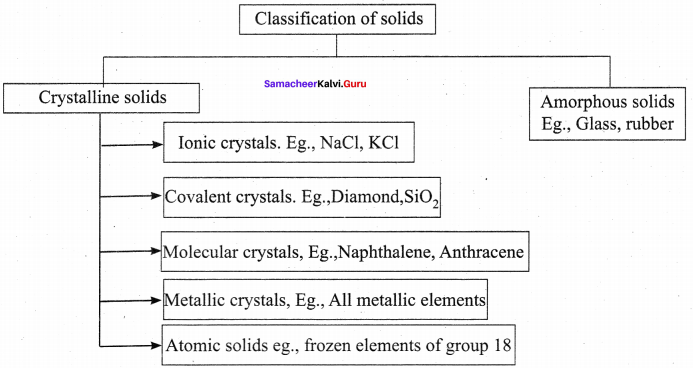Question 3.
What are ionic solids? Give their characteristics.

1. The structural units of an ionic crystals are cations and anions. They are bound together by strong Na electrostatic attractive forces.
2. To maximize the attractive force, cations are surrounded by as many anions as possible and vice versa.
3. Ionic crystals possess definite crystals structure.
4. Many solids are cubic close packed.

Characteristics

1. Ionic solids have high melting points.
2. These solids do not conduct electricity, because the ions are fixed in their lattice positions.
3. They do not conduct electricity in molten state (or) when dissolved in water, because the ions are free to move in the molten state or solution.
4. They are hard as only strong external force can change the relative positions of ions.
5. Example – The arrangement of Na and Cl ions in NaCI crystal.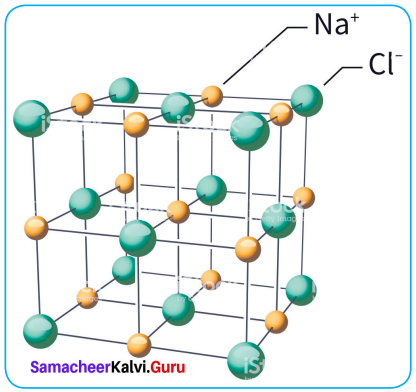Question 4.
What are molecular solids?Explain their classification with suitable examples.
In molecular solids, the constituents are neutral molecules. They are held together by weak vander Waals forces. Generally molecular solids are soft and they do not conduct electricity. These molecular solids are further classified into three types.

1. Non polar molecular solids.
2. Polar molecular solids.
3. Hydrogen bonded molecular solids.

1. Non polar molecular solids

• In this type, molecules are held together by weak dispersion forces or London forces.
• They have low melting points and are usually in liquids or gaseous state at room temperature. Examples – Naphthalene, Anthracene etc.

2. Polar molecular solids

• In this type, molecules formed by polar covalent bonds.
• They are held together by strong dipole-dipole interactions.
• They have higher melting points than the non – polar molecular solids. Examples., solid CO2, solid NH3

3. Hydrogen bonded molecular solids

• The constituents are held together by hydrogen bonds.
• The constituents are held together by hydrogen bonds.
• They are soft solids under room temperature Examples: Solid ice, glucose, urea.

Question 5.
An element crystallizes in a fcc lattice with cell edge of 400 pm. The density of the element is 7 g/cm3. How many atoms one present in 280 g of the element?
Volume of unit cell a3 (a = edge length)
= 400 pm
= (400 x 10-12 m)3
= (400 x 100-10 cm)3 = 64 x 10-24 cm3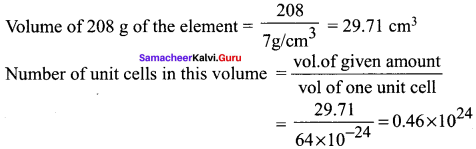Since each f.c.c. unit cell contains 4 atoms therefore,
Total number =4 x 0.46 x 1024,
= 1.84 x 1024 atoms.

Common Errors

Common Errors

1. sc, bcc, fee structures may get confused if they ask in different order.
2. Calculation of atoms at each cube may get confused.
3. Sharing of atoms may get con¬fused.

Rectifications

1. In sc, only simple cube can be drawn, bcc structure is with one dot at centre, fee structure is with sc with six dots at each face.
sc – only one atom, bcc – two atoms, fee – four atoms (we can remember as f and f).
2. Atom at comer is shard by 8 unit cells. Atom at centre is not shared. Atom at face is shared by 2 unit cells. Atom at edge is shared by 4 unit cells.

We hope the Class 12th Samacheer Kalvi Chemistry Solutions Chapter 6 Solid State Book Solutions Answers Guide Solutions provided here help the students to get the best knowledge. Also, students can use our Samacheer Kalvi Class 12th Chemistry Solutions Chapter 6 Solid State Questions and Answers for better practice. You can contact us at any moment by giving comments in the comment section. Keep checking updates by bookmarking our website.